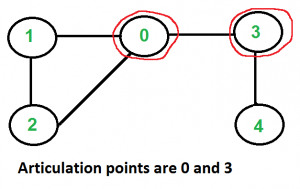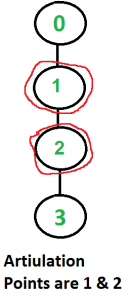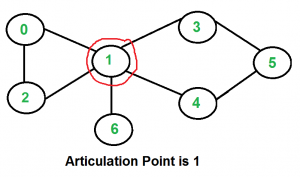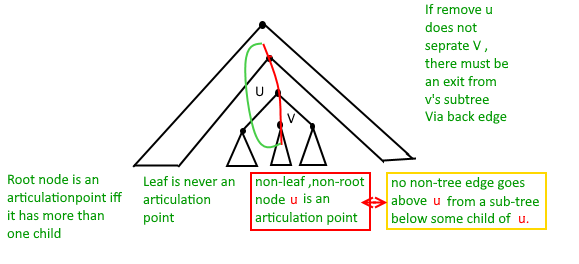# Articulation Points (or Cut Vertices) in a Graph

A vertex in an undirected connected graph is an articulation point (or cut vertex) iff removing it (and edges through it) disconnects the graph. Articulation points represent vulnerabilities in a connected network – single points whose failure would split the network into 2 or more components. They are useful for designing reliable networks.
For a disconnected undirected graph, an articulation point is a vertex removing which increases number of connected components.

Following are some example graphs with articulation points encircled with red color.How to find all articulation points in a given graph?
A simple approach is to one by one remove all vertices and see if removal of a vertex causes disconnected graph. Following are steps of simple approach for connected graph.

1) For every vertex v, do following
…..a) Remove v from graph
..…b) See if the graph remains connected (We can either use BFS or DFS)
…..c) Add v back to the graph

Time complexity of above method is O(V*(V+E)) for a graph represented using adjacency list. Can we do better?

A O(V+E) algorithm to find all Articulation Points (APs)
The idea is to use DFS (Depth First Search). In DFS, we follow vertices in tree form called DFS tree. In DFS tree, a vertex u is parent of another vertex v, if v is discovered by u (obviously v is an adjacent of u in graph). In DFS tree, a vertex u is articulation point if one of the following two conditions is true.
1) u is root of DFS tree and it has at least two children.
2) u is not root of DFS tree and it has a child v such that no vertex in subtree rooted with v has a back edge to one of the ancestors (in DFS tree) of u.

Following figure shows same points as above with one additional point that a leaf in DFS Tree can never be an articulation point.We do DFS traversal of given graph with additional code to find out Articulation Points (APs). In DFS traversal, we maintain a parent[] array where parent[u] stores parent of vertex u. Among the above mentioned two cases, the first case is simple to detect. For every vertex, count children. If currently visited vertex u is root (parent[u] is NIL) and has more than two children, print it.
How to handle second case? The second case is trickier. We maintain an array disc[] to store discovery time of vertices. For every node u, we need to find out the earliest visited vertex (the vertex with minimum discovery time) that can be reached from subtree rooted with u. So we maintain an additional array low[] which is defined as follows.

```low[u] = min(disc[u], disc[w])
where w is an ancestor of u and there is a back edge from
some descendant of u to w.```

Following are C++, Java and Python implementation of Tarjan’s algorithm for finding articulation points.

## C++

 `// A C++ program to find articulation points in an undirected graph ` `#include ` `#include ` `#define NIL -1 ` `using` `namespace` `std; ` ` `  `// A class that represents an undirected graph ` `class` `Graph ` `{ ` `    ``int` `V;    ``// No. of vertices ` `    ``list<``int``> *adj;    ``// A dynamic array of adjacency lists ` `    ``void` `APUtil(``int` `v, ``bool` `visited[], ``int` `disc[], ``int` `low[],  ` `                ``int` `parent[], ``bool` `ap[]); ` `public``: ` `    ``Graph(``int` `V);   ``// Constructor ` `    ``void` `addEdge(``int` `v, ``int` `w);   ``// function to add an edge to graph ` `    ``void` `AP();    ``// prints articulation points ` `}; ` ` `  `Graph::Graph(``int` `V) ` `{ ` `    ``this``->V = V; ` `    ``adj = ``new` `list<``int``>[V]; ` `} ` ` `  `void` `Graph::addEdge(``int` `v, ``int` `w) ` `{ ` `    ``adj[v].push_back(w); ` `    ``adj[w].push_back(v);  ``// Note: the graph is undirected ` `} ` ` `  `// A recursive function that find articulation points using DFS traversal ` `// u --> The vertex to be visited next ` `// visited[] --> keeps tract of visited vertices ` `// disc[] --> Stores discovery times of visited vertices ` `// parent[] --> Stores parent vertices in DFS tree ` `// ap[] --> Store articulation points ` `void` `Graph::APUtil(``int` `u, ``bool` `visited[], ``int` `disc[],  ` `                                      ``int` `low[], ``int` `parent[], ``bool` `ap[]) ` `{ ` `    ``// A static variable is used for simplicity, we can avoid use of static ` `    ``// variable by passing a pointer. ` `    ``static` `int` `time` `= 0; ` ` `  `    ``// Count of children in DFS Tree ` `    ``int` `children = 0; ` ` `  `    ``// Mark the current node as visited ` `    ``visited[u] = ``true``; ` ` `  `    ``// Initialize discovery time and low value ` `    ``disc[u] = low[u] = ++``time``; ` ` `  `    ``// Go through all vertices aadjacent to this ` `    ``list<``int``>::iterator i; ` `    ``for` `(i = adj[u].begin(); i != adj[u].end(); ++i) ` `    ``{ ` `        ``int` `v = *i;  ``// v is current adjacent of u ` ` `  `        ``// If v is not visited yet, then make it a child of u ` `        ``// in DFS tree and recur for it ` `        ``if` `(!visited[v]) ` `        ``{ ` `            ``children++; ` `            ``parent[v] = u; ` `            ``APUtil(v, visited, disc, low, parent, ap); ` ` `  `            ``// Check if the subtree rooted with v has a connection to ` `            ``// one of the ancestors of u ` `            ``low[u]  = min(low[u], low[v]); ` ` `  `            ``// u is an articulation point in following cases ` ` `  `            ``// (1) u is root of DFS tree and has two or more chilren. ` `            ``if` `(parent[u] == NIL && children > 1) ` `               ``ap[u] = ``true``; ` ` `  `            ``// (2) If u is not root and low value of one of its child is more ` `            ``// than discovery value of u. ` `            ``if` `(parent[u] != NIL && low[v] >= disc[u]) ` `               ``ap[u] = ``true``; ` `        ``} ` ` `  `        ``// Update low value of u for parent function calls. ` `        ``else` `if` `(v != parent[u]) ` `            ``low[u]  = min(low[u], disc[v]); ` `    ``} ` `} ` ` `  `// The function to do DFS traversal. It uses recursive function APUtil() ` `void` `Graph::AP() ` `{ ` `    ``// Mark all the vertices as not visited ` `    ``bool` `*visited = ``new` `bool``[V]; ` `    ``int` `*disc = ``new` `int``[V]; ` `    ``int` `*low = ``new` `int``[V]; ` `    ``int` `*parent = ``new` `int``[V]; ` `    ``bool` `*ap = ``new` `bool``[V]; ``// To store articulation points ` ` `  `    ``// Initialize parent and visited, and ap(articulation point) arrays ` `    ``for` `(``int` `i = 0; i < V; i++) ` `    ``{ ` `        ``parent[i] = NIL; ` `        ``visited[i] = ``false``; ` `        ``ap[i] = ``false``; ` `    ``} ` ` `  `    ``// Call the recursive helper function to find articulation points ` `    ``// in DFS tree rooted with vertex 'i' ` `    ``for` `(``int` `i = 0; i < V; i++) ` `        ``if` `(visited[i] == ``false``) ` `            ``APUtil(i, visited, disc, low, parent, ap); ` ` `  `    ``// Now ap[] contains articulation points, print them ` `    ``for` `(``int` `i = 0; i < V; i++) ` `        ``if` `(ap[i] == ``true``) ` `            ``cout << i << ``" "``; ` `} ` ` `  `// Driver program to test above function ` `int` `main() ` `{ ` `    ``// Create graphs given in above diagrams ` `    ``cout << ``"\nArticulation points in first graph \n"``; ` `    ``Graph g1(5); ` `    ``g1.addEdge(1, 0); ` `    ``g1.addEdge(0, 2); ` `    ``g1.addEdge(2, 1); ` `    ``g1.addEdge(0, 3); ` `    ``g1.addEdge(3, 4); ` `    ``g1.AP(); ` ` `  `    ``cout << ``"\nArticulation points in second graph \n"``; ` `    ``Graph g2(4); ` `    ``g2.addEdge(0, 1); ` `    ``g2.addEdge(1, 2); ` `    ``g2.addEdge(2, 3); ` `    ``g2.AP(); ` ` `  `    ``cout << ``"\nArticulation points in third graph \n"``; ` `    ``Graph g3(7); ` `    ``g3.addEdge(0, 1); ` `    ``g3.addEdge(1, 2); ` `    ``g3.addEdge(2, 0); ` `    ``g3.addEdge(1, 3); ` `    ``g3.addEdge(1, 4); ` `    ``g3.addEdge(1, 6); ` `    ``g3.addEdge(3, 5); ` `    ``g3.addEdge(4, 5); ` `    ``g3.AP(); ` ` `  `    ``return` `0; ` `} `

## Java

 `// A Java program to find articulation points in an undirected graph ` `import` `java.io.*; ` `import` `java.util.*; ` `import` `java.util.LinkedList; ` ` `  `// This class represents an undirected graph using adjacency list ` `// representation ` `class` `Graph ` `{ ` `    ``private` `int` `V;   ``// No. of vertices ` ` `  `    ``// Array  of lists for Adjacency List Representation ` `    ``private` `LinkedList adj[]; ` `    ``int` `time = ``0``; ` `    ``static` `final` `int` `NIL = -``1``; ` ` `  `    ``// Constructor ` `    ``Graph(``int` `v) ` `    ``{ ` `        ``V = v; ` `        ``adj = ``new` `LinkedList[v]; ` `        ``for` `(``int` `i=``0``; i The vertex to be visited next ` `    ``// visited[] --> keeps tract of visited vertices ` `    ``// disc[] --> Stores discovery times of visited vertices ` `    ``// parent[] --> Stores parent vertices in DFS tree ` `    ``// ap[] --> Store articulation points ` `    ``void` `APUtil(``int` `u, ``boolean` `visited[], ``int` `disc[], ` `                ``int` `low[], ``int` `parent[], ``boolean` `ap[]) ` `    ``{ ` ` `  `        ``// Count of children in DFS Tree ` `        ``int` `children = ``0``; ` ` `  `        ``// Mark the current node as visited ` `        ``visited[u] = ``true``; ` ` `  `        ``// Initialize discovery time and low value ` `        ``disc[u] = low[u] = ++time; ` ` `  `        ``// Go through all vertices aadjacent to this ` `        ``Iterator i = adj[u].iterator(); ` `        ``while` `(i.hasNext()) ` `        ``{ ` `            ``int` `v = i.next();  ``// v is current adjacent of u ` ` `  `            ``// If v is not visited yet, then make it a child of u ` `            ``// in DFS tree and recur for it ` `            ``if` `(!visited[v]) ` `            ``{ ` `                ``children++; ` `                ``parent[v] = u; ` `                ``APUtil(v, visited, disc, low, parent, ap); ` ` `  `                ``// Check if the subtree rooted with v has a connection to ` `                ``// one of the ancestors of u ` `                ``low[u]  = Math.min(low[u], low[v]); ` ` `  `                ``// u is an articulation point in following cases ` ` `  `                ``// (1) u is root of DFS tree and has two or more chilren. ` `                ``if` `(parent[u] == NIL && children > ``1``) ` `                    ``ap[u] = ``true``; ` ` `  `                ``// (2) If u is not root and low value of one of its child ` `                ``// is more than discovery value of u. ` `                ``if` `(parent[u] != NIL && low[v] >= disc[u]) ` `                    ``ap[u] = ``true``; ` `            ``} ` ` `  `            ``// Update low value of u for parent function calls. ` `            ``else` `if` `(v != parent[u]) ` `                ``low[u]  = Math.min(low[u], disc[v]); ` `        ``} ` `    ``} ` ` `  `    ``// The function to do DFS traversal. It uses recursive function APUtil() ` `    ``void` `AP() ` `    ``{ ` `        ``// Mark all the vertices as not visited ` `        ``boolean` `visited[] = ``new` `boolean``[V]; ` `        ``int` `disc[] = ``new` `int``[V]; ` `        ``int` `low[] = ``new` `int``[V]; ` `        ``int` `parent[] = ``new` `int``[V]; ` `        ``boolean` `ap[] = ``new` `boolean``[V]; ``// To store articulation points ` ` `  `        ``// Initialize parent and visited, and ap(articulation point) ` `        ``// arrays ` `        ``for` `(``int` `i = ``0``; i < V; i++) ` `        ``{ ` `            ``parent[i] = NIL; ` `            ``visited[i] = ``false``; ` `            ``ap[i] = ``false``; ` `        ``} ` ` `  `        ``// Call the recursive helper function to find articulation ` `        ``// points in DFS tree rooted with vertex 'i' ` `        ``for` `(``int` `i = ``0``; i < V; i++) ` `            ``if` `(visited[i] == ``false``) ` `                ``APUtil(i, visited, disc, low, parent, ap); ` ` `  `        ``// Now ap[] contains articulation points, print them ` `        ``for` `(``int` `i = ``0``; i < V; i++) ` `            ``if` `(ap[i] == ``true``) ` `                ``System.out.print(i+``" "``); ` `    ``} ` ` `  `    ``// Driver method ` `    ``public` `static` `void` `main(String args[]) ` `    ``{ ` `        ``// Create graphs given in above diagrams ` `        ``System.out.println(``"Articulation points in first graph "``); ` `        ``Graph g1 = ``new` `Graph(``5``); ` `        ``g1.addEdge(``1``, ``0``); ` `        ``g1.addEdge(``0``, ``2``); ` `        ``g1.addEdge(``2``, ``1``); ` `        ``g1.addEdge(``0``, ``3``); ` `        ``g1.addEdge(``3``, ``4``); ` `        ``g1.AP(); ` `        ``System.out.println(); ` ` `  `        ``System.out.println(``"Articulation points in Second graph"``); ` `        ``Graph g2 = ``new` `Graph(``4``); ` `        ``g2.addEdge(``0``, ``1``); ` `        ``g2.addEdge(``1``, ``2``); ` `        ``g2.addEdge(``2``, ``3``); ` `        ``g2.AP(); ` `        ``System.out.println(); ` ` `  `        ``System.out.println(``"Articulation points in Third graph "``); ` `        ``Graph g3 = ``new` `Graph(``7``); ` `        ``g3.addEdge(``0``, ``1``); ` `        ``g3.addEdge(``1``, ``2``); ` `        ``g3.addEdge(``2``, ``0``); ` `        ``g3.addEdge(``1``, ``3``); ` `        ``g3.addEdge(``1``, ``4``); ` `        ``g3.addEdge(``1``, ``6``); ` `        ``g3.addEdge(``3``, ``5``); ` `        ``g3.addEdge(``4``, ``5``); ` `        ``g3.AP(); ` `    ``} ` `} ` `// This code is contributed by Aakash Hasija `

## Python

 `# Python program to find articulation points in an undirected graph ` `  `  `from` `collections ``import` `defaultdict ` `  `  `#This class represents an undirected graph  ` `#using adjacency list representation ` `class` `Graph: ` `  `  `    ``def` `__init__(``self``,vertices): ` `        ``self``.V``=` `vertices ``#No. of vertices ` `        ``self``.graph ``=` `defaultdict(``list``) ``# default dictionary to store graph ` `        ``self``.Time ``=` `0` `  `  `    ``# function to add an edge to graph ` `    ``def` `addEdge(``self``,u,v): ` `        ``self``.graph[u].append(v) ` `        ``self``.graph[v].append(u) ` `  `  `    ``'''A recursive function that find articulation points  ` `    ``using DFS traversal ` `    ``u --> The vertex to be visited next ` `    ``visited[] --> keeps tract of visited vertices ` `    ``disc[] --> Stores discovery times of visited vertices ` `    ``parent[] --> Stores parent vertices in DFS tree ` `    ``ap[] --> Store articulation points'''` `    ``def` `APUtil(``self``,u, visited, ap, parent, low, disc): ` ` `  `        ``#Count of children in current node  ` `        ``children ``=``0` ` `  `        ``# Mark the current node as visited and print it ` `        ``visited[u]``=` `True` ` `  `        ``# Initialize discovery time and low value ` `        ``disc[u] ``=` `self``.Time ` `        ``low[u] ``=` `self``.Time ` `        ``self``.Time ``+``=` `1` ` `  `        ``#Recur for all the vertices adjacent to this vertex ` `        ``for` `v ``in` `self``.graph[u]: ` `            ``# If v is not visited yet, then make it a child of u ` `            ``# in DFS tree and recur for it ` `            ``if` `visited[v] ``=``=` `False` `: ` `                ``parent[v] ``=` `u ` `                ``children ``+``=` `1` `                ``self``.APUtil(v, visited, ap, parent, low, disc) ` ` `  `                ``# Check if the subtree rooted with v has a connection to ` `                ``# one of the ancestors of u ` `                ``low[u] ``=` `min``(low[u], low[v]) ` ` `  `                ``# u is an articulation point in following cases ` `                ``# (1) u is root of DFS tree and has two or more chilren. ` `                ``if` `parent[u] ``=``=` `-``1` `and` `children > ``1``: ` `                    ``ap[u] ``=` `True` ` `  `                ``#(2) If u is not root and low value of one of its child is more ` `                ``# than discovery value of u. ` `                ``if` `parent[u] !``=` `-``1` `and` `low[v] >``=` `disc[u]: ` `                    ``ap[u] ``=` `True`     `                     `  `                ``# Update low value of u for parent function calls     ` `            ``elif` `v !``=` `parent[u]:  ` `                ``low[u] ``=` `min``(low[u], disc[v]) ` ` `  ` `  `    ``#The function to do DFS traversal. It uses recursive APUtil() ` `    ``def` `AP(``self``): ` `  `  `        ``# Mark all the vertices as not visited  ` `        ``# and Initialize parent and visited,  ` `        ``# and ap(articulation point) arrays ` `        ``visited ``=` `[``False``] ``*` `(``self``.V) ` `        ``disc ``=` `[``float``(``"Inf"``)] ``*` `(``self``.V) ` `        ``low ``=` `[``float``(``"Inf"``)] ``*` `(``self``.V) ` `        ``parent ``=` `[``-``1``] ``*` `(``self``.V) ` `        ``ap ``=` `[``False``] ``*` `(``self``.V) ``#To store articulation points ` ` `  `        ``# Call the recursive helper function ` `        ``# to find articulation points ` `        ``# in DFS tree rooted with vertex 'i' ` `        ``for` `i ``in` `range``(``self``.V): ` `            ``if` `visited[i] ``=``=` `False``: ` `                ``self``.APUtil(i, visited, ap, parent, low, disc) ` ` `  `        ``for` `index, value ``in` `enumerate` `(ap): ` `            ``if` `value ``=``=` `True``: ``print` `index, ` ` `  ` ``# Create a graph given in the above diagram ` `g1 ``=` `Graph(``5``) ` `g1.addEdge(``1``, ``0``) ` `g1.addEdge(``0``, ``2``) ` `g1.addEdge(``2``, ``1``) ` `g1.addEdge(``0``, ``3``) ` `g1.addEdge(``3``, ``4``) ` `  `  `print` `"\nArticulation points in first graph "` `g1.AP() ` ` `  `g2 ``=` `Graph(``4``) ` `g2.addEdge(``0``, ``1``) ` `g2.addEdge(``1``, ``2``) ` `g2.addEdge(``2``, ``3``) ` `print` `"\nArticulation points in second graph "` `g2.AP() ` ` `  `  `  `g3 ``=` `Graph (``7``) ` `g3.addEdge(``0``, ``1``) ` `g3.addEdge(``1``, ``2``) ` `g3.addEdge(``2``, ``0``) ` `g3.addEdge(``1``, ``3``) ` `g3.addEdge(``1``, ``4``) ` `g3.addEdge(``1``, ``6``) ` `g3.addEdge(``3``, ``5``) ` `g3.addEdge(``4``, ``5``) ` `print` `"\nArticulation points in third graph "` `g3.AP() ` ` `  `#This code is contributed by Neelam Yadav `

## C#

 `// A C# program to find articulation  ` `// points in an undirected graph ` `using` `System; ` `using` `System.Collections.Generic; ` ` `  `// This class represents an undirected graph  ` `// using adjacency list representation ` `public` `class` `Graph ` `{ ` `    ``private` `int` `V; ``// No. of vertices ` ` `  `    ``// Array of lists for Adjacency List Representation ` `    ``private` `List<``int``> []adj; ` `    ``int` `time = 0; ` `    ``static` `readonly` `int` `NIL = -1; ` ` `  `    ``// Constructor ` `    ``Graph(``int` `v) ` `    ``{ ` `        ``V = v; ` `        ``adj = ``new` `List<``int``>[v]; ` `        ``for` `(``int` `i = 0; i < v; ++i) ` `            ``adj[i] = ``new` `List<``int``>(); ` `    ``} ` ` `  `    ``// Function to add an edge into the graph ` `    ``void` `addEdge(``int` `v, ``int` `w) ` `    ``{ ` `        ``adj[v].Add(w); ``// Add w to v's list. ` `        ``adj[w].Add(v); ``//Add v to w's list ` `    ``} ` ` `  `    ``// A recursive function that find articulation points using DFS ` `    ``// u --> The vertex to be visited next ` `    ``// visited[] --> keeps tract of visited vertices ` `    ``// disc[] --> Stores discovery times of visited vertices ` `    ``// parent[] --> Stores parent vertices in DFS tree ` `    ``// ap[] --> Store articulation points ` `    ``void` `APUtil(``int` `u, ``bool` `[]visited, ``int` `[]disc, ` `                ``int` `[]low, ``int` `[]parent, ``bool` `[]ap) ` `    ``{ ` ` `  `        ``// Count of children in DFS Tree ` `        ``int` `children = 0; ` ` `  `        ``// Mark the current node as visited ` `        ``visited[u] = ``true``; ` ` `  `        ``// Initialize discovery time and low value ` `        ``disc[u] = low[u] = ++time; ` ` `  `        ``// Go through all vertices aadjacent to this ` `        ``foreach``(``int` `i ``in` `adj[u]) ` `        ``{ ` `            ``int` `v = i; ``// v is current adjacent of u ` ` `  `            ``// If v is not visited yet, then make it a child of u ` `            ``// in DFS tree and recur for it ` `            ``if` `(!visited[v]) ` `            ``{ ` `                ``children++; ` `                ``parent[v] = u; ` `                ``APUtil(v, visited, disc, low, parent, ap); ` ` `  `                ``// Check if the subtree rooted with v has  ` `                ``// a connection to one of the ancestors of u ` `                ``low[u] = Math.Min(low[u], low[v]); ` ` `  `                ``// u is an articulation point in following cases ` ` `  `                ``// (1) u is root of DFS tree and has two or more chilren. ` `                ``if` `(parent[u] == NIL && children > 1) ` `                    ``ap[u] = ``true``; ` ` `  `                ``// (2) If u is not root and low value of one of its child ` `                ``// is more than discovery value of u. ` `                ``if` `(parent[u] != NIL && low[v] >= disc[u]) ` `                    ``ap[u] = ``true``; ` `            ``} ` ` `  `            ``// Update low value of u for parent function calls. ` `            ``else` `if` `(v != parent[u]) ` `                ``low[u] = Math.Min(low[u], disc[v]); ` `        ``} ` `    ``} ` ` `  `    ``// The function to do DFS traversal.  ` `    ``// It uses recursive function APUtil() ` `    ``void` `AP() ` `    ``{ ` `        ``// Mark all the vertices as not visited ` `        ``bool` `[]visited = ``new` `bool``[V]; ` `        ``int` `[]disc = ``new` `int``[V]; ` `        ``int` `[]low = ``new` `int``[V]; ` `        ``int` `[]parent = ``new` `int``[V]; ` `        ``bool` `[]ap = ``new` `bool``[V]; ``// To store articulation points ` ` `  `        ``// Initialize parent and visited, and  ` `        ``// ap(articulation point) arrays ` `        ``for` `(``int` `i = 0; i < V; i++) ` `        ``{ ` `            ``parent[i] = NIL; ` `            ``visited[i] = ``false``; ` `            ``ap[i] = ``false``; ` `        ``} ` ` `  `        ``// Call the recursive helper function to find articulation ` `        ``// points in DFS tree rooted with vertex 'i' ` `        ``for` `(``int` `i = 0; i < V; i++) ` `            ``if` `(visited[i] == ``false``) ` `                ``APUtil(i, visited, disc, low, parent, ap); ` ` `  `        ``// Now ap[] contains articulation points, print them ` `        ``for` `(``int` `i = 0; i < V; i++) ` `            ``if` `(ap[i] == ``true``) ` `                ``Console.Write(i + ``" "``); ` `    ``} ` ` `  `    ``// Driver method ` `    ``public` `static` `void` `Main(String []args) ` `    ``{ ` `        ``// Create graphs given in above diagrams ` `        ``Console.WriteLine(``"Articulation points in first graph "``); ` `        ``Graph g1 = ``new` `Graph(5); ` `        ``g1.addEdge(1, 0); ` `        ``g1.addEdge(0, 2); ` `        ``g1.addEdge(2, 1); ` `        ``g1.addEdge(0, 3); ` `        ``g1.addEdge(3, 4); ` `        ``g1.AP(); ` `        ``Console.WriteLine(); ` ` `  `        ``Console.WriteLine(``"Articulation points in Second graph"``); ` `        ``Graph g2 = ``new` `Graph(4); ` `        ``g2.addEdge(0, 1); ` `        ``g2.addEdge(1, 2); ` `        ``g2.addEdge(2, 3); ` `        ``g2.AP(); ` `        ``Console.WriteLine(); ` ` `  `        ``Console.WriteLine(``"Articulation points in Third graph "``); ` `        ``Graph g3 = ``new` `Graph(7); ` `        ``g3.addEdge(0, 1); ` `        ``g3.addEdge(1, 2); ` `        ``g3.addEdge(2, 0); ` `        ``g3.addEdge(1, 3); ` `        ``g3.addEdge(1, 4); ` `        ``g3.addEdge(1, 6); ` `        ``g3.addEdge(3, 5); ` `        ``g3.addEdge(4, 5); ` `        ``g3.AP(); ` `    ``} ` `} ` ` `  `// This code is contributed by PrinciRaj1992 `

Output:

```Articulation points in first graph
0 3
Articulation points in second graph
1 2
Articulation points in third graph
1```

Time Complexity: The above function is simple DFS with additional arrays. So time complexity is same as DFS which is O(V+E) for adjacency list representation of graph.

Attention reader! Don’t stop learning now. Get hold of all the important DSA concepts with the DSA Self Paced Course at a student-friendly price and become industry ready.

My Personal Notes arrow_drop_up

Improved By : princiraj1992, vthukral94

Article Tags :
Practice Tags :

39

Please write to us at contribute@geeksforgeeks.org to report any issue with the above content.